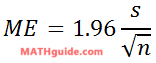Mean, Standard Deviation, and Margin of Error
View the Lesson | MATHguide homepage Updated January 17th, 2022

Given: Sentences were chosen at random from Fritjof Capra's book The Tao of Physics. The number of words in the sentences were counted. This is the result.

 12 8 6 22 4 4 22 11 5 6 12 10 14 6 19 14 8 21 19 19 15 19 13 10
Problem:Use the data above to: a) determine the mean and sample standard deviation, b) calculate a 95% margin of error, c) determine the respective confidence interval.Mean (x̄) =

Sample Standard Deviation (s) =

Margin of Error =

The confidence interval is [, ]